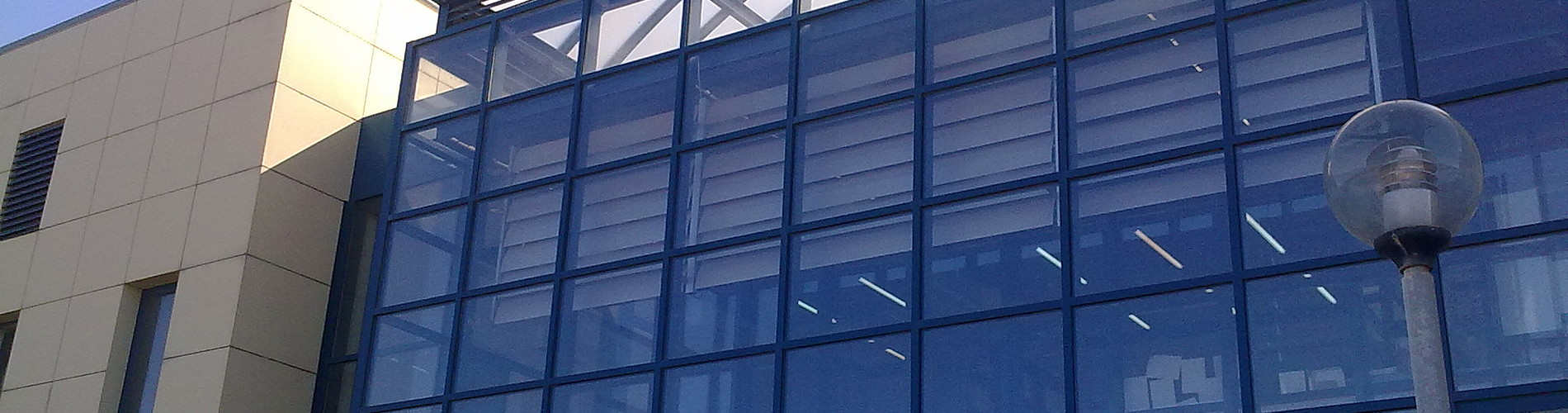School of Chemical and Environmental Engineering

Now offering two distinct diplomas: Chemical Engineering and Environmental Engineering

# Differential and Integral Calculus I

Course Information:

 Course ID: MATH 101 Type of course: Required course Semester: Fall Credits: 5 ΕCTS: 5 Teaching Hours: Th=3, E=1, L=0

Instructor: Associate Professor A. Manoussakis (Faculty Member - EnvEng)

• Thoma's Calculus   (14th Edition)

Eclass:

•  Registration required

Course contents:

• Real variables. Principle of Mathematical induction. Sequences, limits of sequences, recursively defined sequences. Series (Convergence criteria) Functions of one real variable. Limits and continuity of functions. Derivatives. Geometric interpretation of the derivative. Differentials. Applications of the derivative. Definite integrals. Basic theorems of integral calculus. Applications of integration: Αreas between two curves,  volumes and surfaces of solids by revolution, arc length, moments and centroids, hydrostatic pressure.  Exponential Functions. Inverse functions. Hyperbolic functions. Indefinite integral. Integration techniques. Techniques of Integration: Integration by Parts, Trigonometric Integrals,Trigonometric Substitutions,Integration of Rational Functions by Partial Fractions, Improper Integrals. Power series and Taylor series. Applications of series.